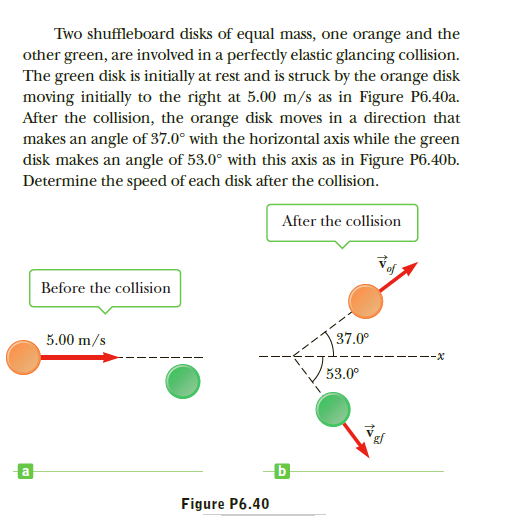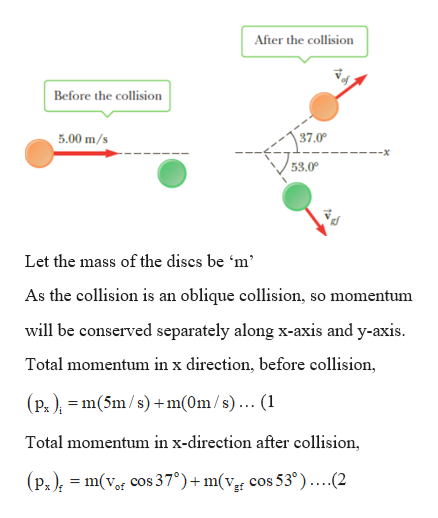# Two shuffleboard disks of equal mass, one orange and theother green, are involved in a perfectly elastic glancing collision.The green disk is initially at rest and is struck by the orange diskmoving initially to the right at 5.00 m/s as in Figure P6.40a.After the collision, the orange disk moves in a direction thatmakes an angle of 37.0° with the horizontal axis while the greendisk makes an angle of 53.0° with this axis as in Figure P6.40b.Determine the speed of each disk after the collision.After the collisionBefore the collision37.0°5.00 m/s--x53.0°Figure P6.40

Question
1 viewshelp_outlineImage TranscriptioncloseTwo shuffleboard disks of equal mass, one orange and the other green, are involved in a perfectly elastic glancing collision. The green disk is initially at rest and is struck by the orange disk moving initially to the right at 5.00 m/s as in Figure P6.40a. After the collision, the orange disk moves in a direction that makes an angle of 37.0° with the horizontal axis while the green disk makes an angle of 53.0° with this axis as in Figure P6.40b. Determine the speed of each disk after the collision. After the collision Before the collision 37.0° 5.00 m/s --x 53.0° Figure P6.40 fullscreen
check_circle

Step 1

Given:

Both the discs (orange and the green) have the same mass.

Step 2

Calculating the speed of each d...help_outlineImage TranscriptioncloseAfter the collision Before the collision 5.00 m/s 37.0 V 53.0° Let the mass of the discs be 'm' As the collision is an oblique collision, so momentum will be conserved separately along x-axis and y-axis. Total momentum in x direction, before collision, (P. ), = m(5m/s) +m(0m/s)... (1 Total momentum in x-direction after collision, (Px); = m(vor cos 37°)+ m(vr cos 53°)....(2 fullscreen

### Want to see the full answer?

See Solution

#### Want to see this answer and more?

Solutions are written by subject experts who are available 24/7. Questions are typically answered within 1 hour.*

See Solution
*Response times may vary by subject and question.
Tagged in

### Collisions